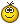# A idea to improve short-range pathfinder's performance

## Recommended Posts

After read short-range path source code deeply.

I found a small problem which maybe cause performance degradation.

The root cause is current algorithm can't discard non-silhouette points.

In GPG2 "Optimizing Points-of-Visibility Pathfinding", it mentioned two optimizations:

"Optimization #1": Only Consider Paths to Silhouette Points

"Optimization #2": Only Consider Paths that Go Around the Corner

So "Optimization #1" may be what we are missing.

Implement "Optimization #1" is very easy, because there are axis-aligned box.

I have written some code in CCmpPathfinder_Vertex.cpp

`// Check that the new vertex is in the right quadrant for the old vertexif (!(vertexes[curr.id].quadOutward & quad)){    // Hack: Always head towards the goal if possible, to avoid missing it if it's    // inside another unit    if (n != GOAL_VERTEX_ID)    {        continue;    }}//------------Modified Begin//If this is an axis-aligned shape, discard non-silhouette points easily.if (vertexes[n].quadInward == QUADRANT_BL)    if ((vertexes[curr.id].p.X >= npos.X && vertexes[curr.id].p.Y >= npos.Y) || (vertexes[curr.id].p.X <= npos.X && vertexes[curr.id].p.Y <= npos.Y))        continue;if (vertexes[n].quadInward == QUADRANT_TR)    if ((vertexes[curr.id].p.X <= npos.X && vertexes[curr.id].p.Y <= npos.Y) || (vertexes[curr.id].p.X >= npos.X && vertexes[curr.id].p.Y >= npos.Y))        continue;if (vertexes[n].quadInward == QUADRANT_TL)    if ((vertexes[curr.id].p.X >= npos.X && vertexes[curr.id].p.Y <= npos.Y) || (vertexes[curr.id].p.X <= npos.X && vertexes[curr.id].p.Y >= npos.Y))        continue;if (vertexes[n].quadInward == QUADRANT_BR)    if ((vertexes[curr.id].p.X <= npos.X && vertexes[curr.id].p.Y >= npos.Y) || (vertexes[curr.id].p.X >= npos.X && vertexes[curr.id].p.Y <= npos.Y))        continue;//------------Modified Endbool visible =    CheckVisibilityLeft(vertexes[curr.id].p, npos, edgesLeft) &&    CheckVisibilityRight(vertexes[curr.id].p, npos, edgesRight) &&    CheckVisibilityBottom(vertexes[curr.id].p, npos, edgesBottom) &&    CheckVisibilityTop(vertexes[curr.id].p, npos, edgesTop) &&    CheckVisibility(vertexes[curr.id].p, npos, edges);`

it seems faster than before.

Has anyone here could review this code?

Sorry for my broken english...

Edited by chansey
•1
##### Share on other sites

There is a little typo line 777 ontinue, instead of continue, apart from that you should come on IRC #0ad-dev.

##### Share on other sites

I believe I tried that when first implementing the algorithm. If I remember correctly, the problem was that sometimes a unit starts from slightly inside a shape or precisely on the edge, and the silhouette-point-detection thing will mean it doesn't see any of the points on that shape at all. That makes the unit pick a very bad path (typically walking to corner of the next nearest building and then back again), which isn't acceptable behaviour.

##### Share on other sites

There is a little typo line 777 ontinue, instead of continue, apart from that you should come on IRC #0ad-dev.

OK, Thanks,

(But there is some time difference.... I will sleep)

##### Share on other sites

a unit starts from slightly inside a shape or precisely on the edge, and the silhouette-point-detection thing will mean it doesn't see any of the points on that shape at all.

"silhouette-point-detection thing will mean it doesn't see any of the points"

why? Fixed point precision problem? (I have not stress test it yet.)

I think if it is a rare problem, can we do some dirty trick (eg: add some delta, if it is a precision problem?) and ensure most of time its behavior correct?

Edited by chansey
##### Share on other sites

I have reproduced the issue which Ykkrosh mentioned.

When the point precisely on the edge, we will get a very bad path.

I modify some code in previous patch.

It seems fixed now.

`//------------Modified Begin//If this is an axis-aligned shape, discard non-silhouette points easily.if (vertexes[n].quadInward == QUADRANT_BL)    if ((vertexes[curr.id].p.X > npos.X && vertexes[curr.id].p.Y > npos.Y) || (vertexes[curr.id].p.X < npos.X && vertexes[curr.id].p.Y < npos.Y))        continue; if (vertexes[n].quadInward == QUADRANT_TR)    if ((vertexes[curr.id].p.X < npos.X && vertexes[curr.id].p.Y < npos.Y) || (vertexes[curr.id].p.X > npos.X && vertexes[curr.id].p.Y > npos.Y))        continue; if (vertexes[n].quadInward == QUADRANT_TL)    if ((vertexes[curr.id].p.X > npos.X && vertexes[curr.id].p.Y < npos.Y) || (vertexes[curr.id].p.X < npos.X && vertexes[curr.id].p.Y > npos.Y))        continue; if (vertexes[n].quadInward == QUADRANT_BR)    if ((vertexes[curr.id].p.X < npos.X && vertexes[curr.id].p.Y > npos.Y) || (vertexes[curr.id].p.X > npos.X && vertexes[curr.id].p.Y < npos.Y))        continue;//------------Modified End`
Edited by chansey
•1
##### Share on other sites

That makes the unit pick a very bad path (typically walking to corner of the next nearest building and then back again), which isn't acceptable behaviour.

Hmm I've seen units doing that with the current implementation, but I don't know if it's caused by the pathfinder.

##### Share on other sites

• 1 year later...

Bumping, might be interesting to reconsider this, i'm sure we could special case the entity the unit is inside in some situations.

•1

## Join the conversation

You can post now and register later. If you have an account, sign in now to post with your account.×   Pasted as rich text.   Paste as plain text instead

Only 75 emoji are allowed.